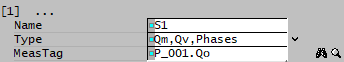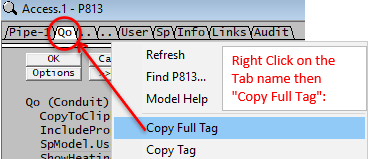# Flow Statistics

Navigation: Models ➔ Statistical Models ➔ Flow Statistics

## Introduction

This model is used in Dynamic Simulation only.

• The Flow Statistics unit operation can be used to collect statistical information of a FLOW variable.Some Valid example of the Flow Stats Measured Tags are: For Pipes/Direct Links: P_001.Qo For Ties: X_001.QFeed For Makeup Source: ProcessWater.QProd• For other variables, for example species flow rate, species concentration, temperature, Level of a tank and so on; use the General Statistics model.
• The Flow Statistics can be reset at Startup by selecting the Statistics tick box on the SysCAD Commands View | Solver Settings - Scenario Tab.

NOTE: User can also use the built in Statistics block in a pipe to collect flow information.

## Data Sections

### Summary of Data Sections

1. FlowStats tab - Contains general information relating to the unit as well as a summary table of each individual statistical blocks.
2. S1 tab - The Flow Stat blocks will be displayed here.
3. Info tab - Contains general settings for the unit and allows the user to include documentation about the unit and create Hyperlinks to external documents.

### Flow Statistics Page

Unit Type: FlowStats The first tab page in the access window will have this name.

### S1: Individual Flow Statistic Data Fields

Flow Statistic is displayed on the Sx pages.

• If "ShowOnePerPage" is NOT selected, then each page displays four Flow Statistic blocks.
• If "ShowOnePerPage" is selected, then each page displays one Flow Statistic block.
Tag (Long/Short) Input / Calc Description/Calculated Variables / Options
[Statistics Block number]
Name Input Name of the statistics block. Eg. Production
Index Display Only available in Build 137 or later. Statistic block index. Useful for sorting in reports
Type Qm Only the total mass flow tags will be visible.
Qm,Qv Total mass and volume flow tags will be visible.
Qm,Phases Total mass flow and phase mass flow tags will be visible.
Qm,Qv,Phases Total mass and volume as well as phase mass flow tags will be visible.
MeasTag Input This is the variable where statistics information will be collected.For Example, when getting a pipe flow rate, enter "P_001.Qo". Tags returned are: "P_001.Qo.Qm", "P_001.Qo.Qv", "P_001.Qo.LQm" and "P_001.Qo.SQm" Other examples include: "TNK_001.QFeed", "X_001.QProd".
ResetStats Button Resets the Statistics information.
Time Calc Time elapsed.
MassFlow / Qm Calc The current mass flow rate.
TotalMass / Mt Calc The total mass passed through the unit operation.
MassFlowAverage / QmAvg Calc The average mass flow over total time.
TimeNoFlow Calc The total time where the unit has no flow.
TimeInvalidFlow / TimeInvalid Calc The time where the unit has a value which is not a number (NAN) (shown as '*').
OnMassFlowAverage / OnQmAvg Calc The average flow over (Total time – Time with Zero Flow).
MinMassFlow / QmMin Calc The minimum flow over Total time.
MaxMassFlow / QmMax Calc The maximum flow over Total time.
VolFlow / Qv Calc The volumetric flowrate.
TotalVol / Vt Calc The total volume passed through the unit operation.
VolFlowAverage / QvAvg Calc The average volumetric flowrate over total time.
SolidsMassFlow / SQm Calc The current Solids Flowrate.
SolidMass / SMt Calc The total Solids passed through the unit operation.
SolidMassFlowAvg / SQmAvg Calc The average solids flowrate over total time.
LiquidMassFlow / LQm Calc The current Liquid Flowrate.
LiquidMass / LMt Calc The total Liquids passed through the unit operation.
LiquidMassFlowAvg / LQmAvg Calc The average Liquid flowrate over total time.
Delete Button This allows the user to Delete the current individual Control block. Please note that there is no 'Undo'!
MoveUp Button This allows the user to increase the Priority of the current individual Control block. For example, if the current Control block is number 3, the user can change it to 2 or 1 by clicking on this button once or twice.
MoveDown Button This allows the user to decrease the Priority of the current individual Control block. For example, if there are 3 Control blocks in the unit and the current Control block is number 1, the user can change it to 2 or 3 by clicking on this button once or twice.

## Adding this Model to a Project

Insert into Configuration file

Sort either by DLL or Group.

 DLL: ControlDyn.dll → Units/Links → Statistics: Flow or Group: General → Units/Links → Statistics: Flow